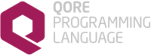Qore Programming Language Reference Manual 1.19.1
Searching...
No Matches
SQL Constants

## Variables

const Qore::SQL::BLOB = "blob"
for binding BLOB values

const Qore::SQL::CLOB = "clob"
for binding CLOB values

const Qore::SQL::DATE = "date"
for binding date/time values

const Qore::SQL::DECIMAL = "number"
for binding decimal values as a number More...

const Qore::SQL::NUMBER = "number"
for binding number values as a number More...

const Qore::SQL::NUMERIC = "number"
for binding numeric values as a number More...

const Qore::SQL::RESULTSET = "resultset"
For binding result set placeholders. More...

const Qore::SQL::VARCHAR = "string"
for binding string values

SQL constants

## ◆ DECIMAL

 const Qore::SQL::DECIMAL = "number"

for binding decimal values as a number

Since
Qore 0.8.6 the value of this constant is `"number"` instead of `"string"`

## ◆ NUMBER

 const Qore::SQL::NUMBER = "number"

for binding number values as a number

Since
Qore 0.8.6 the value of this constant is `"number"` instead of `"string"`

## ◆ NUMERIC

 const Qore::SQL::NUMERIC = "number"

for binding numeric values as a number

Since
Qore 0.8.6 the value of this constant is `"number"` instead of `"string"`

## ◆ RESULTSET

 const Qore::SQL::RESULTSET = "resultset"

For binding result set placeholders.

For SQLStatement output values in supported DBI drivers

Since
Qore 0.8.13